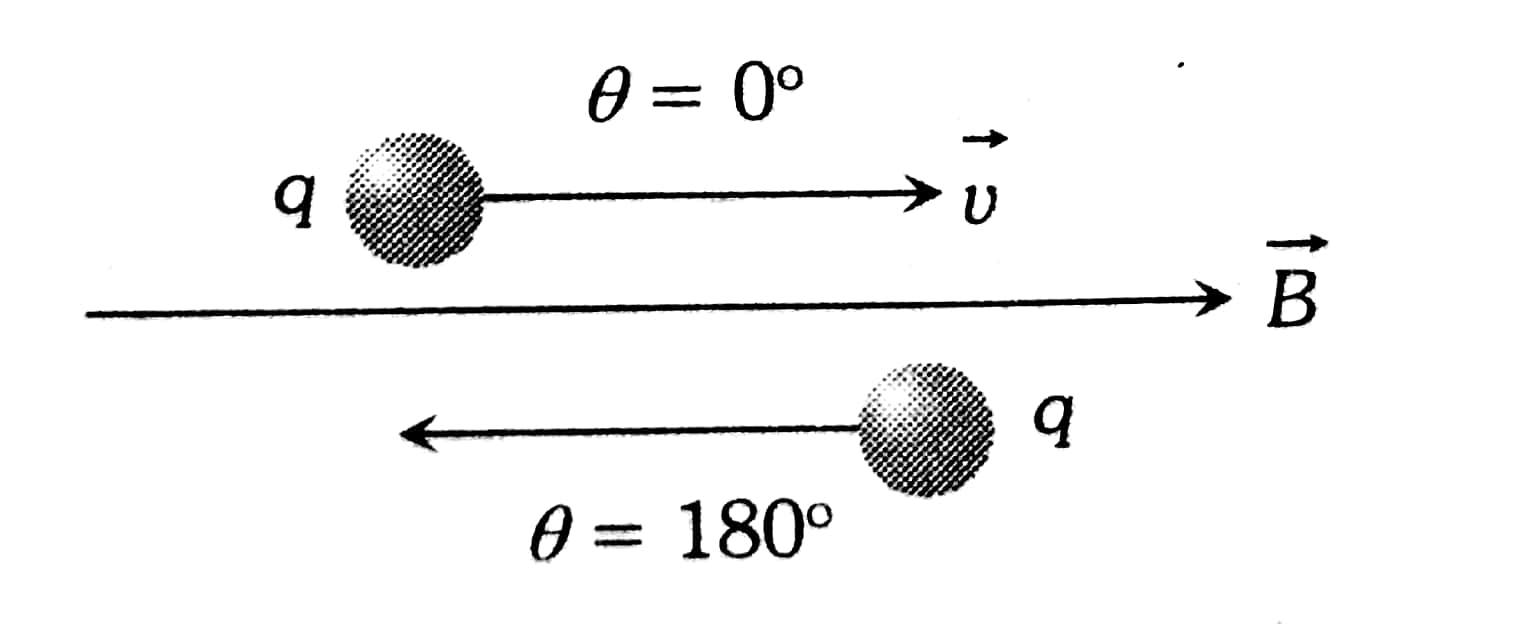#### In a region, steady and uniform electric and magnetic fields are present. These two fields are parallel to each other. A charged particle is released from rest in this region. The path of the particle will be a Option 1) circle Option 2) helix Option 3) straight line Option 4) ellipse.As we learnt in

Path of charge particle If θ = 0° or θ = 180° -

Trajectory of the particle is a straight line

i.e.-F=0

- whereinMagnetic field exerts a force = eBvsin= eBvsin 0 = 0

Electric field exerts force along a straight line The path of charged particle will be a straight line.

Option 1)

circle

Incorrect option

Option 2)

helix

Incorrect option

Option 3)

straight line

Correct option

Option 4)

ellipse.

Incorrect option

#### Plabita